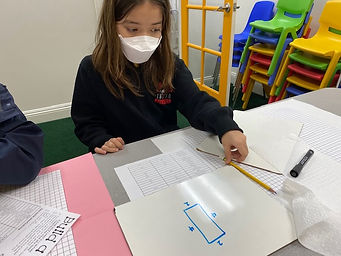Ms. Dawn

Target 1​

Lesson Type:

Continuation

Measurment

:

Relationships

Understand the relationship that exists between area and perimeter.

1:

Understand that figures with the same area can have different perimeters (and vice versa).

2:

Identify patterns and strategies involved in working with area and perimeter.

4th

Vocabulary:

Area, Perimeter, Figures, Formula

Activities:

1. Students were given either th perimeter or area and needed to find the other.

2. Students drew pictures on the whiteboard to help them solve both area or perimeter.

3. After finding the information needed, Students started to create their blue print of a house design.Home Exploration

Guiding Questions:Absent Students:

Target 2

:

1:

Identify the correct arithmetic processes based on the information presented in word problems.

2:

Find the key words in word problems that indicate the correct arithmetic process to use: Addition: add, altogether, both, combined, in all, plus, sum, total; Subtraction: difference, fewer, how many/much more, left, less, minus, remains; Multiplication: times, product, each, twice; and Division: how many in each, divided by, quotient, goes into, split evenly.

3:

Find and use the needed information in a word problem to solve.

4th

Vocabulary:

CUBE, Addition, Subtraction, Division, Multiplication, Operation, Multi-step

Activities:

1. Students went around the room answering multi-step word problems. They used startegies learned, such as the "CUBE" method. They also needed to find key words that would help them understand what operation they needed to use in order to solve the problem.

2. Students showed their work in specific boxes. Once they got the answer, they needed to walk to find the answer that led them to the next problem.Home Exploration

Guiding Questions:Target 3

:

Vocabulary:

Activities:Home Exploration